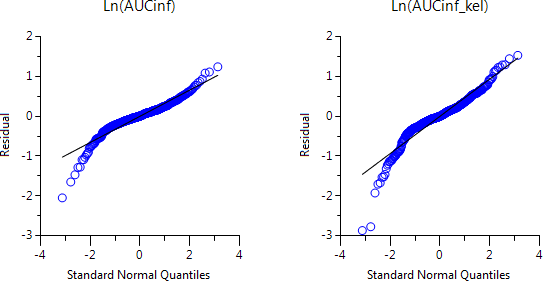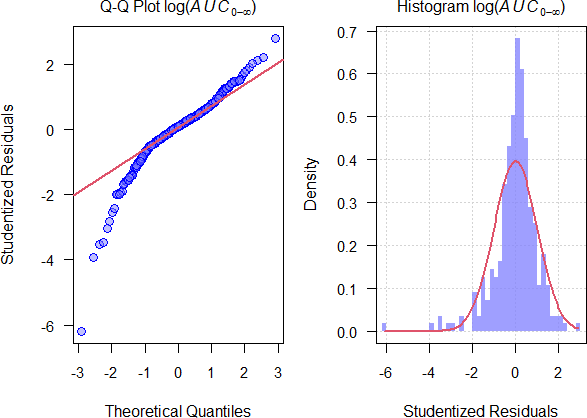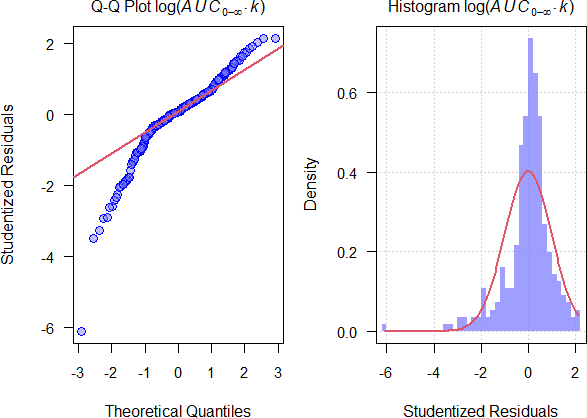Helmut
★★★Vienna, Austria,
2021-09-30 23:31
(268 d 04:42 ago)

Posting: # 22608
Views: 1,526

## AUC · k for variable inter-occasion CL [NCA / SHAM]

Dear all,

let’s recap the basic mass balance equation of PK: $$\small{F\cdot D=V\cdot k\,\cdot\int_{0}^{\infty}C(t)\,dt=CL\cdot AUC_{0-\infty}}\tag{1}$$ We assess BE by comparative BA, i.e., $$\small{\frac{F_{\textrm{T}}}{F_{\textrm{R}}}\approx \frac{AUC_{\textrm{T}}}{AUC_{\textrm{R}}}}\tag{2}$$ That’s only part of the story because – based on $$\small{(1)}$$ – actually $$\small{AUC_{\textrm{T}}=\frac{F_\textrm{T}\cdot D_\textrm{T}}{CL}\;\land\;AUC_{\textrm{R}}=\frac{F_\textrm{R}\cdot D_\textrm{R}}{CL}}\tag{3}$$ Since (except for Health Canada and in exceptional cases for the EMA) a dose-correction is not acceptable, we have to assume that the true contents equal the declared ones and further $$\small{D_\textrm{T}\equiv D_\textrm{R}}\tag{4}$$ This allows us to eliminate the doses from $$\small{(3)}$$; however we still have to assume no inter-occasion variability of Clearances ($$\small{CL=\textrm{const}}$$) in order to arrive at $$\small{(2)}$$.
Great but is that true? If we have to deal with a Highly Variable Drug, the high variability is an intrinsic property of the drug itself (not the formulation). In BE were are interested in detecting potential differences of formulations, right? Since we ignored the – possibly unequal – Clearances, all unexplained variability goes straight into the resi­dual error, results in a high CV and hence, a wide confidence interval. In other words, the formulation is punished for a crime that Clearance has committed.
Can we do anything against it – apart from reference-scaling? We know that$$\small{k=CL\big{/}V}\tag{5}$$ In a cross-over design the volume of distribution of subjects likely shows limited inter-occasion variability. In a parallel design we can minimize its variability by carefully selecting subjects with similar anthropometric properties (body weight, age, etc.) in the groups. Therefore, we can drop the volume of distribution and approximate the ef­fect of $$\small{CL}$$ by $$\small{k}$$. This leads to $$\small{\frac{F_{\textrm{T}}}{F_{\textrm{R}}}\sim \frac{AUC_{\textrm{T}}\cdot k_{\textrm{T}}}{AUC_{\textrm{R}}\cdot k_{\textrm{R}}}}\tag{6}$$ A variant of $$\small{(6)}$$ – using $$\small{t_{1/2}}$$ instead of $$\small{k}$$ – was proposed already in the dark ages* by Wagner.1 Later work by an author of the FDA (!)2 was ignored as well. Hey, for 20+ years! A recent paper3 demonstrated its usefulness in extensive simulations.

Abstract
Aim: To quantify the utility of a terminal-phase adjusted area under the concentration curve method in increasing the probability of a correct and conclusive outcome of a bioequivalence (BE) trial for highly variable drugs when clearance (CL) varies more than the volume of distribution (V).
Methods: Data from a large population of subjects were generated with variability in CL and V, and used to simulate a two-period, two-sequence crossover BE trial. The 90% confidence interval for formulation comparison was determined following BE assessment using the area under the concentration curve (AUC) ratio test, and the proposed terminal-phase adjusted AUC ratio test. An outcome of bioequivalent, non-bioequivalent or inconclusive was then assigned according to predefined BE limits.
Results: When CL is more variable than V, the proposed approach would enhance the probability of correctly assigning bioequivalent or non-bioequivalent and reduce the risk of an inconclusive trial. For a hypothetical drug with between-subject variability of 35% for CL and 10% for V, when the true test-reference ratio of bioavailability is 1.15, a cross-over study of n=14 subjects analyzed by the proposed method would have 80% or 20% probability of claiming bioequivalent or non-bioequivalent, compared to 22%, 46% or 32% probability of claiming bioequivalent, non-bioequivalent or inconclusive using the standard AUC ratio test.
Conclusions: The terminal-phase adjusted AUC ratio test represents a simple and readily applicable approach to enhance the BE assessment of drug products when CL varies more than V.

I ❤️ the idea. When Abdallah’s paper2 was published, I tried it retrospectively in a couple of my studies. Worked mostly, and if not, it was a HVDP, where the variability is caused by the formulation (e.g., gastric-resistant diclo­fenac).

• When the portmanteau word ‘bioavailability’ (of ‘biological’ and ‘availability’) was in the future. Try to search for papers prior to ~1975 with ‘bioavailability’. You will be surprised.

1. Wagner JG. Method of Estimating Relative Absorption of a Drug in a Series of Clinical Studies in Which Blood Levels Are Measured After Single and/or Multiple Doses. J Pharm Sci. 1967; 56(5): 652–3. doi:10.1002/jps.2600560527.
2. Abdallah HY. An area correction method to reduce intrasubject variability in bioequivalence studies. J Pharm Pharm Sci. 1998; 1(2): 60–5.Open access.
3. Lucas AJ, Ogungbenro K, Yang S, Aarons L. Chen C. Evaluation of area under the concentration curve adjusted by the terminal-phase as a metric to reduce the impact of variability in bioequivalence testing. Br J Clin Pharmacol. Early View 16 July 2021. doi:10.1111/bcp.14986.

Dif-tor heh smusma 🖖Helmut SchützThe quality of responses received is directly proportional to the quality of the question asked. 🚮
Science Quotes
PharmCat
★

Russia,
2021-10-01 00:15
(268 d 03:59 ago)

@ Helmut
Posting: # 22609
Views: 1,418

## AUC · k for variable inter-occasion CL

Hey!
Helmut,
Sounds great. One problem ... I don't think that our regulators will be able to understand this, or there will be a desire and dare to change the foundations. Also, I think NLME modeling can be applied.
Helmut
★★★Vienna, Austria,
2021-10-01 11:18
(267 d 16:56 ago)

@ PharmCat
Posting: # 22610
Views: 1,386

## Changing the regulations: Hope dies last.

Hi PharmCat,

» I don't think that our regulators will be able to understand this, …

Well, the majority of regulators is not stupid.That’s basic PK and $$\small{CL=\textrm{const}}$$ is a pretty strong assumption, which might be outright false. Then the entire concept is built on sand: Studies are substantially larger than necessary, exposing innocent subjects to nasty drugs.

» … or there will be a desire and dare to change the foundations.

Correct. However, the FDA1 gives in its regulatory definitions of BE ‘rate and extent of absorption’ without mentioning any PK metrics, the EMA2 ‘BE with the reference medicinal product […] demonstrated by appropriate bioavail­ability studies’. Only the WHO3 gives specifically AUC for the extent of absorption. Too lazy to check other jurisdictions.
Somehow it reminds me on the comparison of $$\small{AUC_{t_\textrm{last}}}$$, which is known to be biased if $$\small{t_\textrm{last,T}\neq t_\textrm{last,R}}$$. Nobody gives a shit. Remember the endless story of the inflated Type I Error in SABE? For HVDs (not HVDPs) probably we could counteract the high variability, use ABE with fixed limits, and all is good.
Of course, it would mean changing the guidelines.

Abdallah (FDA/CDER):

It is recommended that area correction be attempted in bioequivalence studies of drugs where high intrasubject variability in clearance is known or suspected. […] The value of this approach in regulatory decision making remains to be determined.

Lucas et al.:

Performance of the AUC·k ratio test […] indicate that the regulators should consider the method for its potential utility in assessing HVDs and lessening unnecessary drug exposure in BE trials.

» Also, I think NLME modeling can be applied.

Hhm, can you elaborate?

1. CFR 21–320.23(b)(1). 2021.
2. Directive 2001/83/EC, Article 10(2)(b). 2001.
3. TRS 992, Annex 6. 2017.

P.S.: $$\small{C_\textrm{max}}$$ is a lousy metric for comparing rates of absorption, since it is composite of $$\small{k_\textrm{a}}$$ and $$\small{AUC_{0-\infty}}$$. Lots of publications… What about: $$\small{\frac{F_\textrm{T}}{F_\textrm{R}}\sim \frac{C_\textrm{max,T}\big{/}\left(AUC_{0-\infty,\textrm{T}}\cdot k_\textrm{T} \right)}{C_\textrm{max,R}\big{/}\left(AUC_{0-\infty,\textrm{R}}\cdot k_\textrm{R} \right)}}$$ Deserves a paper.

Dif-tor heh smusma 🖖Helmut SchützThe quality of responses received is directly proportional to the quality of the question asked. 🚮
Science Quotes
d_labes
★★★

Berlin, Germany,
2021-10-01 13:52
(267 d 14:21 ago)

@ Helmut
Posting: # 22611
Views: 1,243

## AUC * k

Dear both,

what do you think is a reasonable assumption about the distribution of the metric AUC*k?
Do we have to throw away our evaluation of BE studies assuming log-normal distri of the metrics AUC and/or Cmax?

Regards,

Detlew
Helmut
★★★Vienna, Austria,
2021-10-01 17:01
(267 d 11:13 ago)

@ d_labes
Posting: # 22612
Views: 1,261

## AUC * k

Dear Detlew,

» what do you think is a reasonable assumption about the distribution of the metric AUC*k?

Since both are lognormal, their ratio should be lognormal as well. I trust here Martin; will meet him in the evening and ask again. Furthermore, the distribution of values per se is not important, only the residual error.

One of my 4-period full replicate studies (143 subjects, Method A)» Do we have to throw away our evaluation of BE studies assuming log-normal distri of the metrics AUC and/or Cmax?

Not at all – if they passed.Too bad if they failed and would have passed with AUC·k…

Dif-tor heh smusma 🖖Helmut SchützThe quality of responses received is directly proportional to the quality of the question asked. 🚮
Science Quotes
d_labes
★★★

Berlin, Germany,
2021-10-02 10:55
(266 d 17:19 ago)

@ Helmut
Posting: # 22614
Views: 1,157

## AUC * k

Dear Helmut,

» Since both are lognormal, their ratio should be lognormal as well. I trust here Martin; will meet him in the evening and ask again.
» Furthermore, the distribution of values per se is not important, only the residual error.

Correct.

» One of my 4-period full replicate studies (143 subjects, Method A)
»Looks not too bad for a log-normal.

Regards,

Detlew
Helmut
★★★Vienna, Austria,
2021-10-03 20:29
(265 d 07:45 ago)

@ d_labes
Posting: # 22616
Views: 1,145

## Ratio/product of two lognormals

Dear Detlew,

» » » what do you think is a reasonable assumption about the distribution of the metric AUC*k?
» » Since both are lognormal, their ratio should be lognormal as well. I trust here Martin; will meet him in the evening and ask again.

Answer: The difference of two normal distributions is normal distributed. The ratio and product of two lognormal distributions are lognormal distributed.
Furthermore, the unit of AUC·k is mass/volume.n   <- 2501 x   <- seq(0.01, 2.5, length.out = n) a   <- dlnorm(x = x, meanlog = log(1),    sdlog = sqrt(log(0.15^2 + 1))) b   <- dlnorm(x = x, meanlog = log(0.95), sdlog = sqrt(log(0.30^2 + 1))) c   <- a / b d   <- a * b clr <- c("#FF808080", "#8080FF80", "#FF80FF80") dev.new(width = 4.5, height = 4.5, record = TRUE) op <- par(no.readonly = TRUE) par(mar = c(4, 0, 0, 0), cex.axis = 0.9) plot(x = x, y = a, type = "n", xlab = expression(mu[T]/mu[R]),      ylab = "", ylim = range(a, b, c), axes = FALSE) axis(1) polygon(x = c(x, rev(x)), y = c(rep(0, n), rev(a)), col = clr, border = NA) polygon(x = c(x, rev(x)), y = c(rep(0, n), rev(b)), col = clr, border = NA) polygon(x = c(x, rev(x)), y = c(rep(0, n), rev(c)), col = clr, border = NA) legend("topright", lwd = 5, seg.len = 2, col = clr, cex = 0.8,        legend = c(expression(italic(a)*" = dlnorm(log(1), CV 0.15)"),                   expression(italic(b)*" = dlnorm(log(0.95), CV 0.30)"),                   expression(italic(c==a/b)))) plot(x = x, y = a, type = "n", xlab = expression(mu[T]/mu[R]),      ylab = "", ylim = range(a, b, d), axes = FALSE) axis(1) polygon(x = c(x, rev(x)), y = c(rep(0, n), rev(a)), col = clr, border = NA) polygon(x = c(x, rev(x)), y = c(rep(0, n), rev(b)), col = clr, border = NA) polygon(x = c(x, rev(x)), y = c(rep(0, n), rev(d)), col = clr, border = NA) legend("topright", lwd = 5, seg.len = 2, col = clr, cex = 0.8,        legend = c(expression(italic(a)*" = dlnorm(log(1), CV 0.15)"),                   expression(italic(b)*" = dlnorm(log(0.95), CV 0.30)"),                   expression(italic(d==a %.% b)))) par(op)

Dif-tor heh smusma 🖖Helmut SchützThe quality of responses received is directly proportional to the quality of the question asked. 🚮
Science Quotes
Helmut
★★★Vienna, Austria,
2021-10-04 12:05
(264 d 16:08 ago)

@ d_labes
Posting: # 22618
Views: 1,115

## Not for HVDPs?

Dear Detlew,

» » One of my 4-period full replicate studies (143 subjects, Method A)
»
» Looks not too bad for a log-normal.

More details… The reference formulation in this study was terrible; CVwR twice of CVwT, many subjects with low AUCs after R. Distributions of studentized model residuals heavy-tailed. Since CVwT <30%, this was a HVDP and not a HVD. Adjusting the AUC would make things worse.
90% CIs (Method B, Satterthwaite’s df):
AUC0–∞:    111.17 – 123.79%
AUC0–∞·k: 113.89 – 132.70%Dif-tor heh smusma 🖖Helmut SchützThe quality of responses received is directly proportional to the quality of the question asked. 🚮
Science Quotes
mittyri
★★

Russia,
2021-10-04 14:22
(264 d 13:51 ago)

@ Helmut
Posting: # 22619
Views: 1,085

Dear Helmut,

» More details… The reference formulation in this study was terrible; CVwR twice of CVwT, many subjects with low AUCs after R. Since CVwT <30%, this was a HVDP and not a HVD. Adjusting the AUC would make things worse.

now try something with horrible enterohepatic cirulationKind regards,
Mittyri
Helmut
★★★Vienna, Austria,
2021-10-04 16:10
(264 d 12:03 ago)

@ mittyri
Posting: # 22620
Views: 1,036

## Oh dear!

Hi mittyri,

» now try something with horrible enterohepatic cirulationThe masochistic part of my psyche is limited.*

However, the method is not a Swiss army-knife. I would never perform a pivotal replicate study without a pilot in order to see what’s going on.

• OK, OK, my curiosity won.
Highly lipophylic drug, ~50% excreted unchanged into the bile, documented enterohepatic recycling. No idea why this study was performed in a 2×2×2 crossover in two groups (ended in a deficiency about a ‘significant group-by-treatment interaction’…). Why not a 4-period full re­pli­cate in one group?
115 eligible subjects, target metrics Cmax, AUC0–72. t½ 10.6 h, recalculated residual AUC ~15% (2.2% – 86%!). CVintra of AUC0–∞ larger than the one of Cmax.$$\small{\begin{array}{lrcccccr} \hline \;\;\;\;\;\;\;\text{PK metric} & \text{PE}\;\; & \text{90% CL}_\textrm{lower} & \text{90% CL}_\textrm{upper} & \text{width} & CV_\textrm{intra} & CV_\textrm{inter} & n\;\;\\\hline AUC_{0-\infty} & 93.35 & 85.38 & 102.07 & 16.68 & 42.54 & 45.11 & 74\\ AUC_{0-\infty}\cdot k & 93.67 & 86.43 & 101.51 & 15.08 & 38.04 & 39.58 & 60\\ C_\textrm{max} & 95.55 & 87.92 & 103.84 & 15.92 & 39.47 & 36.23 & 64\\ C_\textrm{max}/AUC_{0-\infty} & 102.35 & 96.78 & 108.23 & 11.45 & 25.98 & 29.61 & 30\\ C_\textrm{max}/AUC_{0-\infty}\cdot k & 102.69 & 91.81 & 114.86 & 23.05 & 54.74 & 65.53 & 114\\\hline \end{array}}$$ $$\small{n}$$: Sample size for 2×2×2 crossover, T/R-ratio 0.95, ≥80% power.
Lots of limitations: Poor estimation of $$\small{\lambda_\textrm{z}}$$ (n = 3: 218, n = 4: 12), likely not the terminal phase (reported t½ 20 – 36 h), in 35.7% of cases extrapolated AUC >20%.
Required sample sizes: $$\small{AUC_{0-\infty}\cdot k}$$ vs $$\small{AUC_{0-\infty}}$$ –19%, $$\small{C_\textrm{max}/AUC_{0-\infty}}$$ vs $$\small{C_\textrm{max}}$$ –53%.
$$\small{C_\textrm{max}/AUC_{0-\infty}\cdot k}$$ is awful. Why?

Dif-tor heh smusma 🖖Helmut SchützThe quality of responses received is directly proportional to the quality of the question asked. 🚮
Science Quotes
PharmCat
★

Russia,
2021-10-01 18:48
(267 d 09:26 ago)

@ Helmut
Posting: # 22613
Views: 1,277

## Changing the regulations: Hope dies last.

» Hhm, can you elaborate?

these are my thoughts, I did not support them with any deep search ...
$$C = C_0 * e^{-k_e * t}$$ => $$C = \frac{D}{V_d} * e^{-k_e * t}$$
Model:
$$C = \frac{aX + \epsilon_2}{V_d} * e^{-bZ * t} * \epsilon_1$$
$$\epsilon_1 \sim N(0, \sigma_1^2); \epsilon_2 \sim N(0, \sigma_2^2)$$
It can be solved for $$a, V_d, b, \sigma_1^2, \sigma_2^2$$ and modified for extravascular model.

Something described here: Korkmaz, Selcuk & Orman, Mehmet. (2012). The Use of Nonlinear Mixed Effects Models in Bioequivalence Studies: A Real Data Application. Open Access Scientific Reports. 1-11

However, I think terminal-phase adjusted area under the concentration curve method is something between the classic and NLME approaches. And both of them make corrections for variation from elimination.Ing. Helmut Schütz